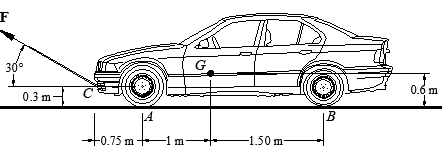# 8.5) The automobile has a mass of 1.7 Mg and center of mass at G. Take ?s = 0.3. Determine the...

## Question:

The automobile has a mass of 1.7 Mg and center of mass at G. Take ?s = 0.3. Determine the towing force F required to move the car if the back brakes are locked, and the front wheels are free to roll.## Force

The property of a body with the help of which body tends to change its shape size and the nature of the motion or the position it is in is called force. Mathematically it can be defined as the product of the mass of the body and the acceleration of the body with which the body is moving.

Given Data:

• The mass of the car is: {eq}m = 1.7\;{\rm{Mg}} {/eq}.
• The coefficient of static friction is: {eq}{\mu _s} = 0.3 {/eq}.
• The angle at...

Become a Study.com member to unlock this answer! Create your account